# Capacitance of Three Phase Overhead Line

## Capacitance of Three Phase Overhead Line:

In a Capacitance of Three Phase Overhead Line, the capacitance of each conductor is considered instead of capacitance from conductor to conductor. Here, again two cases arise viz., symmetrical spacing and unsym­metrical spacing.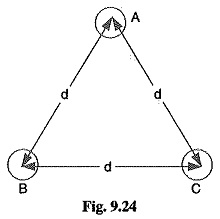### 1.Symmetrical Spacing:

Fig. 9.24 shows the three con­ductors A, B and C of the 3-phase overhead transmission line having charges QA, QB and QC per metre length respectively. Let the conductors be equidistant (d metres) from each other. We shall find the capacitance from line conductor to neutral in this symmetrically spaced line. Referring to Fig. 9.24, overall potential difference between conductor A and infinite neutral plane is given by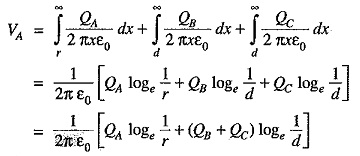Assuming Balanced supply, we have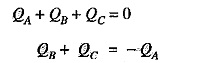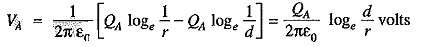Capacitance of conductor A w.r.t neutral,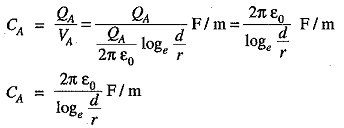Note that this equation is identical to capacitance to neutral for two-wire line. Derived in a similar manner, the expressions for capacitance are the same for conductors B and C.

### 2.Unsymmetrical Spacing:

Fig. 9.25 shows a 3-phase transposed line having unsymmetrical spacing. Let us assume balanced conditions i.e. QA + QB + QC = 0.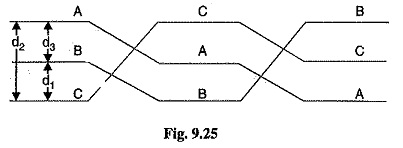Considering all the three sections of the transposed line for phase A,

Potential of 1st position,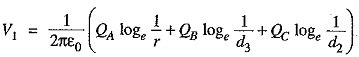Potential of 2nd position,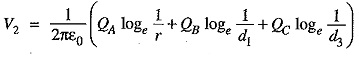Potential of 3rd position,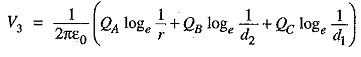Average voltage on condutor A is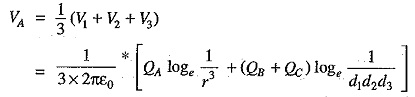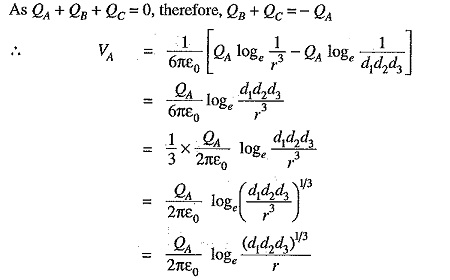Capacitance from conductor to neutral is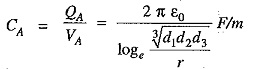Updated: May 23, 2018 — 11:43 pm